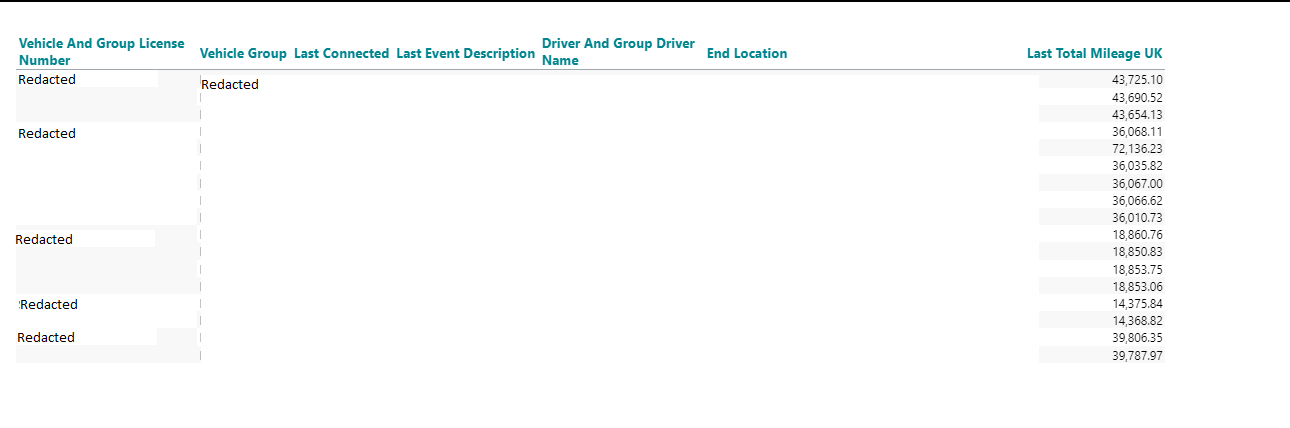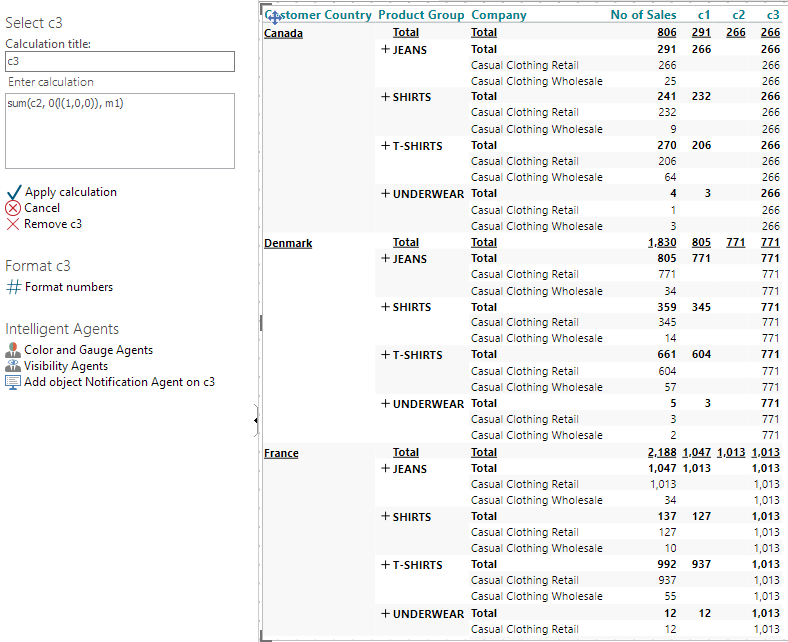# Calculate max value

Hi,

I have a crosstab where I need  to calculate the max value per licence number.

These are the dimension members being used:Might be a simple calculation, but I'm simply stocked at this stage.

Does anyone has a suggestion on how this can be achieved?

Thank you!

/Steffi

• Hi Steppi,

I have a solution, but I wouldn't label it 'simple'. :-)

From your screenshot, it seems like you have 6 dimensions on the vertical axis (and only one measure). This would require 6 calculations to get to desired result.

Added as calculated columns (c1 to c6) these would be:

`c1: max(d1, all(c), m1)c2: max(c1, all(c), m1)c3: max(c2, all(c), m1)c4: max(c3, all(c), m1)c5: max(c4, all(c), m1)c6: sum(c5, 0(l(1,0,0,0,0,0)), m1)`

In my example, below, I have reproduced it with 3 dimensions on the vertical axis. C1 and C2 is as described above, while C3 is as you see it in the screenshot.

I included the subtotals for clarity, but they can be hidden.BR / Ole

• Hi Ole,

with some small modifications, this worked perfectly fine for me.

Thank you!

Br. Steffi